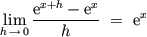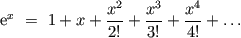1. If f(x)=e<sup>x</sup> why is f' equal to e<sup>x</sup>?2. ### Related Discussions:

3. When you take the derivative of a function and evaluate it at a point, you obtain the rate of change, or slope, at that point. The graph of e<sup>x</sup> has a slope of one at 0. Thus, the derivative of e<sup>x</sup> is e<sup>x</sup> because if you evaluate it at 0, you obtain e<sup>0</sup> = 1. I've read that the e<sup>x</sup> graph is actually defined by having a slope of 1 at x = 0. I'm sure someone can give a better answer, but that's how I know it.4.Originally Posted by thyristor
If f(x)=e<sup>x</sup> why is f' equal to e<sup>x</sup>?
Because5. Also note that, in Jane's description, the factor e<sup>x</sup> pulls out of the limit, so that you can conclude that the derivative of e<sup>x</sup> is:

e<sup>x</sup> lim<sub>h->0</sub> (e<sup>h</sup>-1)/h

So then the fact that e<sup>x</sup> is its own derivative boils down to the fact that the above limit is 1.6.Originally Posted by JaneBennetOriginally Posted by thyristor
If f(x)=e<sup>x</sup> why is f' equal to e<sup>x</sup>?
BecauseI don't understand how you can see that that as an answer to my question. When I asked my question I wanted to be answered something containing the very special definition of e. The thing I'm curious about is why not all functions a<sup>b</sup> have the derivata a<sup>b</sup>.7. You want to do it another way? TryNow differentiate term by term. What do you get?8. You can also look at it from the opposite side thyristor, think of the logarithm ln(x) = ∫ dt/t where we integrate from 1 to x. Now a quick look at the chain rule shows that (e<sup>x</sup>)' = e<sup>x</sup>.

However, if you think of what happens to a logarithm when you change the basis of that logarithm you will see that you introduce a constant that you just can't get rid of. This constant is what ruins this property for the other exponents. In fact, e is that number such that ∫ dt/t where we integrate from 1 to e is equal to 1 (so the constant is no problem)9.Originally Posted by JaneBennet
You want to do it another way? TryNow differentiate term. What do you get?
I see, it's the same
1+x+x<sup>2</sup>/2!+x<sup>3</sup>/3!...
Quite neat.10. Thanks for the help everybody :-DBookmarks
 Posting Permissions
 You may not post new threads You may not post replies You may not post attachments You may not edit your posts   BB code is On Smilies are On [IMG] code is On [VIDEO] code is On HTML code is Off Trackbacks are Off Pingbacks are Off Refbacks are On Terms of Use Agreement